# Selina Concise Mathematics Class 10 ICSE Solutions Chapter 1 GST (Goods and Services Tax)

## Selina Concise Mathematics Class 10 ICSE Solutions Chapter 1 GST (Goods and Services Tax)

Students should practice Goods & Service Tax (GST) – CS Executive Tax Laws MCQ Questions with Answers based on the latest syllabus.

Selina Publishers Concise Mathematics Class 10 ICSE Solutions Chapter 1 GST (Goods and Services Tax)

### GST Exercise 1A – Selina Concise Mathematics Class 10 ICSE Solutions

Question 1.
For the following transaction within Delhi, fill in the blanks to find the amount of bill :
MRP = Rs. 12,000, Discount % = 30%, GST = 18%
Discount =
Selling price (discounted value) =
CGST =
SGST =
IGST =
Amount of Bill =
Solution:
MRP = Rs. 12,000, Discount % = 30%, GST= 18%
Discount = 30% of 12,000= $$\frac{30}{100}$$ × 12000 = Rs. 3600
Selling price (discounted value) = 12000 – 3600
= Rs. 8400
CGST= 9% of 8400 = Rs. 756
SGST= 9% of 8400 = Rs. 756
IGST= 0
Amount of Bill = Selling price + CGST + SGST = 8400 + 756 + 756 = Rs. 9912

Question 2.
For the following transaction from Delhi to Jaipur, fill in the blanks to find the amount of bill :
MRP = Rs. 50,000, Discount % = 20%, GST = 28%
Discount =
Selling price (discounted value) =
CGST =
SGST =
IGST =
Amount of Bill =
Solution:
MRP = Rs. 50,000, Discount % = 20%, GST= 28%
Discount = 20% of 50,000 = $$\frac{20}{100}$$ × 50,000 = Rs. 10,000
Selling price (discounted value) = 50,000 – 10,000
= Rs. 40,000
CGST = 0
SGST = 0
IGST = 28% of 40,000 = $$\frac{28}{100}$$ × 40,000 = Rs. 11,200
Amount of Bill = Selling price + IGST
= 40,000 + 11,200
= Rs. 51,200

Question 3.
A computer mechanic in Delhi charges repairing cost from five different persons A, B, C, D and E with certain discounts. The repairing costs and the corresponding discounts are as given below :If the rate of GST is 18%, find the total money (including GST) received by the mechanic.
Solution:
Name of the person Repairing cost (in Rs.) Discount % Discount Selling price CGST (9%) SGST (9%)The total money (including GST) received by the mechanic is 18,820 + 1693.8 + 1693.8
= Rs. 22,207.6

Question 4.
Find the amount of bill for the following intra-state transaction of goods/services. The GST rate is 5%.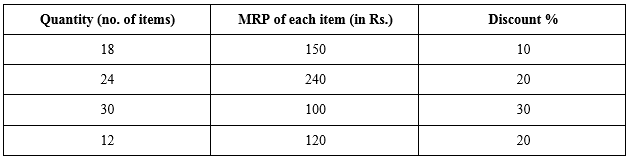Solution: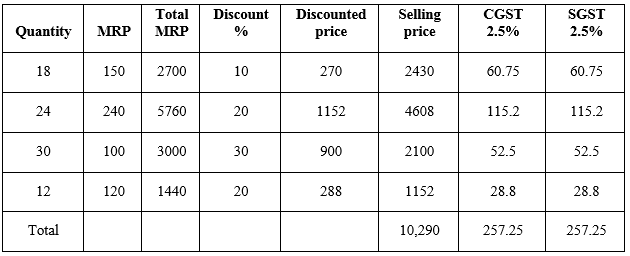Amount of bill = Selling price + GST
= 10,290 + 257.25 + 257.25
= Rs. 10,804.5

Question 5.
Find the amount of bill for the following inter-state transaction of goods/services. The GST rate is 18%.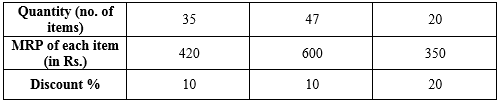Solution:Amount of bill = Selling price + CGST + SGST
= 44,210 + 3978.9 + 3978.9
= Rs. 52,167.8

Question 6.
Find the amount of bill for the following intra-state transaction of goods/services.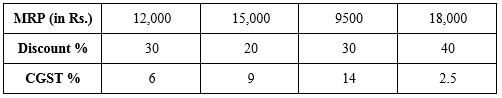Solution:Amount of bill = Selling price + CGST + SGST
= 37,850 + 2785 + 2785
= Rs. 43,420

Question 7.
Find the amount of bill for the following inter-state transaction of goods/services.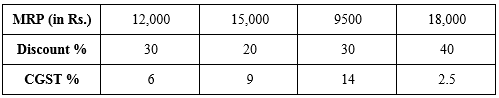Solution: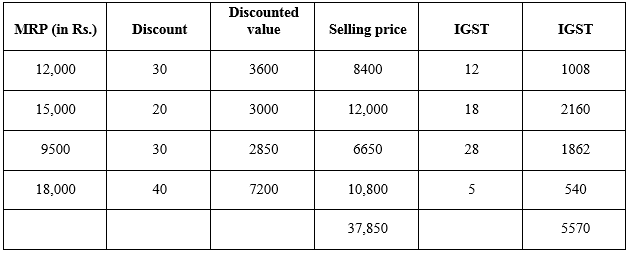Amount of bill = Selling price + GST
= 37,850 + 5570
= Rs. 43,420

Question 8.
A dealer in Mumbai supplied an item at the following prices to a dealer in Delhi. Find the total amount of the bill.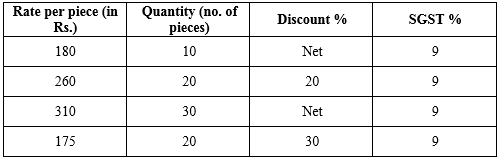Solution:Amount of bill = Selling price + IGST
= 17,710 + 3187.8
= Rs. 20,897.8

Question 9.
National Trading Company, Meerut (UP) made the supply of the following goods/services to Samarth Traders, Noida (UP). Find the total amount of bill if the rate of GST = 12%Solution:Amount of bill = Selling price + SGST + CGST
= 17,220 + 2066.4
= Rs. 19,286.4

Question 10.
M/s Ram Traders, Delhi, provided the following services to M/s Geeta Trading Company in Agra (UP). Find the amount of bill :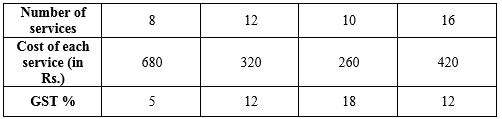Solution: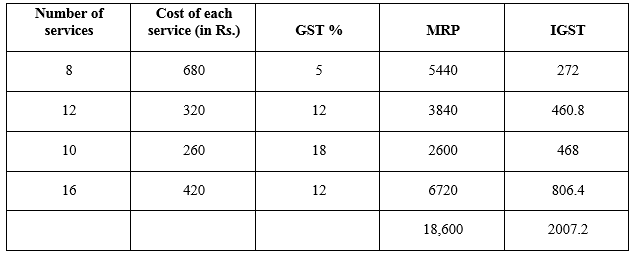Amount of bill = Selling price + IGST
= 18,600 + 2007.2
= Rs. 20,607.2

Question 11.
For the following, find the amount of bill data :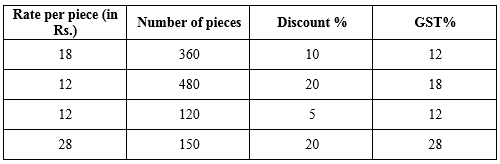Solution:Amount of bill = Selling price + GST
= 15,168 + 2634.24
= Rs. 17,802.24

Question 12.
The tax invoice of a telecom service in Meerut shows cost of services provided by it as Rs. 750. If the GST rate is 18%, find the amount of the bill.
Solution:
According to the question,
GST = 18% of 750
= $$\frac{18}{100}$$ × 750 = Rs. 135
The amount of bill = 750 + 135 = Rs. 885

Question 13.
Mr. Pankaj took Health Insurance Policy for his family and paid Rs. 900 as SGST. Find the total annual premium paid by him for this policy, rate of GST being 18%.
Solution:
Let the total annual premium paid by Mr. Pankaj be Rs. x. According to the question, 18% of x= SGST + CGST 18% of x= 1800 SGST= CGST $$\frac{18}{100}$$ × x = 1800
x = Rs. 10,000

Question 14.
Mr. Malik went on a tour to Goa. He took a room in a hotel for two days at the rate of Rs. 5000 per day. On the same day, his friend John also joined him. Hotel provided an extra bed charging Rs. 1000 per day for the bed. How much GST, at the rate of 28% is charged by the hotel in the bill to Mr. Malik for both the days?
Solution:
According to the question,
The amount of bill = 5000 × 2 + 1000 + 1000
= 10,000 + 2000
= Rs. 12,000
GST= 28% of 12,000
= $$\frac{28}{100}$$ × 12,000 = Rs. 3360
GST charged by Mr. Malik Rs. 3360.

Question 15.
Asharaf went to see a movie. He wanted to purchase a movie ticket for Rs. 80. As the ticket for Rs. 80 was not available, he purchased a ticket for Rs. 120 of upper class. How much extra GST did he pay for the ticket? (GST for a ticket below Rs. 100 is 18% and GST for a ticket above Rs. 100 is 28%)
Solution:
According to the question,
GST on ticket of Rs. 80 = 18% of 80= $$\frac{18}{100}$$ × 80 = Rs. 14.40
GST on ticket of Rs. 120 = 28% of 120= $$\frac{18}{100}$$ × 120 = Rs. 33.60
Difference between both GST
= 33.60 – 14.40
= Rs. 19.20

### GST Exercise 1B- Selina Concise Mathematics Class 10 ICSE Solutions

Question 1.
Fill in the blanks :
When the goods/services are sold for Rs. 15,000 under intra-state transaction from station A to station B and the rate of GST is 12%.
As per GST System
S.P. at station A =
CGST = 6% of 15,000 =
SGST = 6% of 15,000 =
C.P. at station B =
If profit = Rs. 5000
S.P. at station B =
Now the same goods/services are moved under inter-state transaction from station B to station C and the rate of tax is 12%.
GST =
C.P. at station C =
Solution:
When the goods/services are sold for Rs. 15,000 under intra-state transaction from station A to station B and the rate of GST is 12%.
As per GST System
S.P. at station A = Rs. 15,000
CGST = 6% of 15,000 = Rs. 900
SGST = 6% of 15,000 = Rs. 900
C.P. at station B = Rs. 15,000
If profit = Rs. 5000
S.P. at station B = 15,000 + 5000
= Rs. 20,000
Now the same goods/services are moved under inter-state transaction from station B to station C and the b rate of tax is 12%.
GST = 12% of 20,000
= Rs. 2400
C.P. at station C = Rs. 20,000

Question 2.
Goods/services are sold from Agra (U.P.) to Kanpur (U.P.) for Rs. 20,000 and then from Kanpur to Jaipur (Rajasthan). If the rate of GST is 18% and the profit made at Kanpur is Rs. 5000, find:

• the net GST payable by the dealer at Kanpur.
• the cost of goods/services at Jaipur.

Solution:
When the product is sold from Agra to Kanpur (intra-state transaction)
For the dealer in Agra :
S. P. in Agra = Rs. 20,000
CGST = 9% of Rs. 20,000 = $$\frac{9}{100}$$ × 20,000 = 1800
SGST = 9% of Rs. 20,000 = $$\frac{9}{100}$$ × 20,000 = 1800
When product is sold from Kanpur to Jaipur (inter-state transaction)
For the dealer in Kanpur
Input-tax credit = 1800 + 1800 = Rs. 3600
C. P. = Rs. 20,000 and Profit = Rs. 5000
S.P. = 20,000 + 5000 = Rs. 25,000
IGST = 18% of 25,000 = Rs. 4500

Net GST paid by the dealer at Kanpur
= Output GST – Input GST
= 4500 – 3600
= Rs. 900

The cost of goods/services at Jaipur
= S. P. in Agra + IGST
= 25,000 + 18% of 25000
= 25,000 + 4500
= Rs. 29,500

Question 3.
Goods/services are sold from Kota (Rajasthan) to Mumbai for Rs. 20,000 and then from Mumbai to Pune. If the rate of GST is 12% and the profit made at Mumbai is Rs. 5000; find the net GST paid at Pune, if the dealer at Pune is the end-user.
Solution:
For the dealer in Mumbai (inter-state transaction)
CP = Rs. 20,000
IGST = 12% of Rs. 20,000 = $$\frac{12}{100}$$ × 20,000 = Rs. 2400
Profit = Rs. 5000
SP = Rs. 25,000
For the dealer in Pune (intra-state transaction)
CP = Rs. 25,000
CGST = 6% of 25,000 = Rs. 1500
SGST = 6% of 25,000 = Rs. 1500
GST payable by the end user at Pune = 1500 + 1500= Rs. 3000

Question 4.
A is a dealer in Banaras (U.P.). he supplies goods/services worth Rs. 8000 to a dealer B in Agra (U.P.). Dealer B, in turn, supplies the same goods/services to dealer C in Patna (Bihar) at a profit of Rs. 1200. Find the input and output taxes for the dealer C under GST system; if the rate of GST is 18% and C does not sell his goods/services further.
Solution:
For the dealer A (intra-state transaction)
SP= Rs. 8,000

For the dealer B (intra-state transaction)
CP = Rs. 8,000
CGST = 9% of 8,000 = Rs. 720
SGST = 9% of 8,000 = Rs. 720
Profit = Rs. 1,200
SP = Rs. 9,200

For the dealer C (inter-state transaction)
CP = Rs. 9,200
IGST = 18 % of Rs. 9,200 = $$\frac{18}{100}$$ × 9,200 = Rs. 1656
Input Tax = Rs. 1,656
Since, the dealer in Patna does not sell the product.
Output GST (tax on sale) = Rs. 0

Question 5.
A is a dealer in Meerut (U.P.). He supplies goods/services, worth Rs. 15,000 to a dealer B in Ratlam (M.P.). Dealer B, in turn, supplies the same goods/services to dealer C in Jabalpur (M.P.) at a profit of Rs. 3000. If rate of tax (under GST system) is 18%, find

• The cost of goods/services to the dealer C in Jabalpur.
• Net tax payable by dealer B.

Solution:
For A (case of inter-state transaction)
S.P. in Meerut = Rs. 15,000

For B (case of inter-state transaction)
C.P. = Rs. 15,000
IGST = 18% of 15,000 = $$\frac{18}{100}$$ × 15,000 = Rs. 2700
Output tax for B = Rs. 2,700
C.P. in Ratlam = Rs. 2700 and profit = Rs. 3000
S.P. in Ratlam = 15,000 + 3000 = Rs. 18,000
Output tax for B =
For C (case of intra-state transaction)
C.P. = Rs. 18,000
CGST = 9% of 18,000 = $$\frac{9}{100}$$ × 18,000 = Rs. 1620
SGST = $$\frac{9}{100}$$ × 18,000 = Rs. 1620
Net GST payable by the dealer B
= Output tax – Input tax
= 1620 + 1620 – 2700
= Rs. 540
C.P. for the dealer C in Jabalpur
= S.P. for the dealer in Ratlam + GST
= 18,000 + 1620 + 1620
= Rs. 21,240

Question 6.
A dealer X in Hapur (U.P.) supplies goods/services, worth Rs. 50,000 to some other dealer Y in the same city. Now the dealer Y supplies the same goods/services to dealer Z in Calcutta at a profit of Rs. 20,000. Find

• Output and input taxes for the dealer Y
• Net GST payable by dealer Y.

The rate of GST at each stage is 28%
Solution:
For the dealer X (intra-state transaction)
SP= Rs. 50,000

For the dealer Y (intra-state transaction)
CP = Rs. 50,000
CGST = 14% of 50,000= Rs. 7,000
SGST = 14% of 50,000= Rs. 7,000
Input tax for dealer Y = Rs. 14,000
Profit = Rs. 20,000
SP = Rs. 70,000

For the dealer Z (inter-state transaction)
CP = Rs. 70,000
IGST = 28 % of Rs. 70,000 = $$\frac{28}{100}$$ × 70,000 = Rs. 19,600
∴ Input Tax = Rs. 19,600 which is the output tax for dealer Y.

Net GST payable for Y
= Output tax for Y – Input tax for Y
= 19,600 – 14,000
= Rs. 5600

Question 7.
Consultancy services, worth Rs. 50,000, are transferred from Delhi to Calcutta at the rate of GST 18% and then from Calcutta toNainital (with profit = Rs. 20,000) at the same rate of GST. Find the output tax at

• Delhi
• Calcutta
• Nainital

Solution:
Output tax in Delhi (interstate) :
IGST = 9% of 50,000 = Rs. 9000
Output tax in Delhi = Rs. 9000

Output tax in Calcutta :
C.P. in Calcutta = Rs. 50,000 and Profit
= Rs. 20,000
S.P. in Calcutta = 50,000 + 20,000
= Rs. 70,000
IGST = 18% of 70,000 = Rs. 12,600
Output tax in Calcutta = Rs. 12,600
Since, the dealer in Nainital does not sell the product.
Output GST (tax on sale) = Rs. 0

Question 8.
For a dealer A, the list price of an article is Rs. 9000, which he sells to dealer B at some lower price. Further, dealer B sells the same article to a customer at its list price. If the rate of GST is 18% and dealer B paid a tax, under GST, equal to Rs. 324 to the government, find the amount (inclusive of GST) paid by dealer B.
Solution:
Let A sells to dealer B at Rs. x lower price.
According to the question,
Net Tax paid by dealer B is
⇒ Out put tax – Input Tax = Rs. 324
⇒ 18% of 9000 – 18% of (9000 – x) = 324
⇒ 1620 – 1620 + 18% of x = 324
⇒ 18% of x = 324
⇒ x= 1800
Hence, selling price of B = 9000 – 1800 = Rs. 7200
The amount (inclusive of GST) paid by dealer B
= 7200 + 18% of 7200
= 7200 + 1296
= Rs. 8496

Question 9.
The marked price of an article is Rs. 6000. A wholesaler sells it to a dealer at 20% discount. The dealer further sells the article to a customer at a discount of 10% on the marked price. If the rate of GST at each stage is 18%, find the amount of tax (under GST) paid by the dealer to the government.
Solution:
Initial marked price by manufacturer A is Rs. 6000
B bought the T.V. at a discount of 20%.
Cost price of B = 80% of 6000 = Rs. 4800 ….(i)
GST paid by B for purchase = 18% of 4800 = Rs. 864 ….(ii)
B sells T.V. at discount of 10% of market Price
Selling price for B = 6000 – 10% of 6000 = Rs. 5400 …(iii)
GST charged by B on selling of T.V. = 18% of 5400 = Rs. 972 …(iv)
GST paid by B to the government
= GST charged on selling price – GST paid against purchase price
= 972 – 864
= Rs. 108

Question 10.
A is a manufacturer of T.V. sets in Delhi. He manufacturers a particular brand of T.V. set and marks it at Rs. 75,000. He then sells this T.V. set to a wholesaler B in Punjab at a discount of 30%. The wholesaler B raises the marked price of the T.V. set bought by 30% and then sells it to dealer C in Delhi. If the rate of GST = 5% find tax (under GST) paid by wholesaler B to the government.
Solution:
Initial marked price by manufacturer A is Rs. 75,000
B bought the T.V. at a discount of 30%.
Cost price of B = 70% of 75,000 = Rs. 52,500 ….(i)
GST paid by B for purchase = 5% of 52,500 = Rs. 2625 ….(ii)
B sells T.V. by increasing marked price by 30%.
Selling price for B = 75,000 + 30% of 75,000 = Rs. 97,500 …(iii)
GST charged by B on selling of T.V. = 5% of 97,500
= Rs. 4875 …(iv)
GST paid by B to the government
= GST charged on selling price – GST paid against purchase price
= 4875 – 2625
= Rs. 2250

Question 11.
For a trader, marked price of a refrigerator = Rs. 15,680 inclusive of GST at the rate of 12% on the marked price. Gagan, a customer for this refrigerator, asks the trader to reduce the marked price of the refrigerator to such extend that its reduced price plus GST on it is equal to marked price of the refrigerator. Find the required reduction.
Solution:
Let the marked price be Rs. x.
x + 12% of x = 15,680
1.12x = 15,680
x = Rs. 14,000
Initial marked price = Rs. 14,000
Let Gagan asked for priced reduction of Rs. y.
New price = 14,000 – y
GST on new price = 12% of (14,000 – y)
According to the question,
14,000 – y + 0.12(14,000 – y) = 14,000
-1.12y + 1680 = 0
y = 1500
Required reduction in price is Rs. 1500.